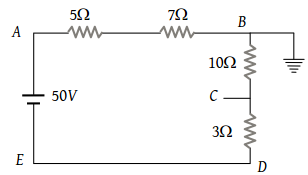In the circuit shown, the point ‘B’ is earthed. The potential at the point ‘A’ is(1) 14 V

(2) 24 V

(3) 26 V

(4) 50 V

(2) Current in the given circuit $i=\frac{50}{\left(5+7+10+3\right)}=2A$

Potential difference between A and B ${V}_{A}-{V}_{B}=2×12$

${V}_{A}-0=24V$${V}_{A}=24\text{\hspace{0.17em}}V$

Difficulty Level:

• 10%
• 56%
• 12%
• 25%